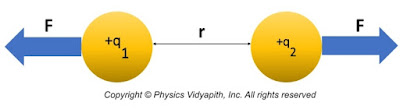### Coulomb′s Law and Applications

Coulomb’s Law: This law was first published by French physicist Charles-Augustin de Coulomb in the year 1785. According to Coulomb’s Law-
The Electric force acting between the two point charges is directly proportional to product of magnitude of the two charges and inversely proportional to square of distance between these two charges. The electric force always acts along the line joining the charges.Coulomb's force between the two charges
Let us consider two positive charges whose magnitude $q_{1}$ and $q_{2}$ are placed at a distance $‘r’$. According to Coulomb’s Law (magnitude only):

$F\propto q_{1}q_{2} \qquad(1)$

$F\propto \frac{1}{r^{2}} \qquad(2)$

From equation$(1)$ and equation$(2)$, we can write as:

$F\propto \frac{q_{1}q_{2}}{r^{2}} \qquad(3)$

$F=\frac{1}{4\pi \varepsilon K} \frac{q_{1}q_{2}}{r^{2}} \qquad(4)$

Where
$\epsilon$= Permittivity of any medium,
$K$ = Dielectric constant

For air and vacuum:
$\epsilon= \epsilon_{0}$ and $K=1$

So Coulomb’s Law for Air and Vacuum:

$F=\frac{1}{4\pi \varepsilon_{0}}\frac{q_{1}q_{2}}{r^{2}} \qquad(5)$

Where $\varepsilon _{0}=8.0854 \times 10^{-12} \:\: C^{2}N^{-1}m^{-2}$

Then, the value of $\frac{1}{4\pi \varepsilon_{0}}=9\times10^{9} N-m^{2}/C^{2}$ So, From Equation$(5)$

$F=9\times 10^{9} \frac{q_{1}q_{2}}{r^{2}}$

This is the equation of Coulomb's Law that is applicable for the medium of air or vacuum. The above equation of Coulomb Law shows only the magnitude value of electrostatic force.

Properties of coulomb's law:-

There are the following properties of coulomb's law:-

1. Coulomb force is action and reaction pair and follows Newton's third law.

2. Coulomb force is a conservative force.

3. Coulomb force is central force i.e. it is always acting along the line joining between two charges.

4. If the net force is zero then momentum will be conserved.

5. If the center of mass at rest and momentum is conserved then it follows the mass conservation law.

6. The force between two charges is independent of the presence or absence of other charges but the net force increase on that particular charge.

Limitation of Coulomb's Law:

1. It is not applicable to moving charges i.e. Coulomb's Law applies to static charges and charges must be stationary with respect to each other.

2. It is applicable to charges of regular and smooth shape. It is very difficult to apply to irregular shapes.

3. The charges must not overlap for example they must be point charges.

4. This charge can not be directly applicable to calculate the charge on big planets.

Application of Coulomb's Law:

1. Coulomb's law is used to calculate the distance between the charges.

2. Coulomb's law is used to calculate the force between the charges.

3. Coulomb's law is used to calculate the force on a point due to the presence of several point charges. It is also known as the Superposition theorem.

**What is $1$ Coulomb's?

Answer: When two unit charges are placed in a vacuum at one meter apart then the force acting between charges is $9\times10^{9}$ $N$.This force is known as 1 Coulomb.